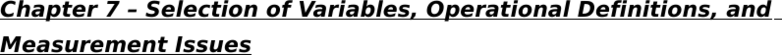Textbook Notes (290,000)
CA (170,000)
U of G (10,000)
PSYC (3,000)
PSYC 2360 (100)
Chapter 7

# PSYC 2360 Chapter Notes - Chapter 7: Birth Weight, Operational Definition, Dependent And Independent Variables

Department
Psychology
Course Code
PSYC 2360
Professor
Carol Anne Hendry
Chapter
7

Page:
of 2Chapter 7 Selection of Variables, Operational Definitions, and
Measurement Issues
- An independent variable is the variable in a study we manipulate
- A dependent variable is the variable being measured
Levels of the independent variable - The number of conditions for a
specific independent variable
e.g., low birth weight & normal birth weight
- 4 different types of dependent variables:
1) Behavioural measure - A measure to investigate a person’s
behaviours
e.g., how often a student attends a certain class
2) Attitudinal measure - A measure assessing a person’s attitudes on
the topic
e.g., whether a student has a positive or negative attitude toward a
certain class
3) Cognitive measure - A measure of one’s mental ability or knowledge
of a topic
e.g., how well a student knows about the materials in a certain class
4) Physiological measure - A measure that is biological in nature
e.g., heart rate, pulse, blood pressure
- 3 most common ways a dependent variable is assessed
1) Perfect correct - Average of correct responses to overall responses
represented as a percentage
e.g., the percentage of students who are correct in answering a certain
question
2) Frequency of responding - A sum of the number of times a person or
a group responds to a question
e.g., number of participants responded to a comedian by laughing
3) Degree of response - A measure of intensity of a response
e.g., how hard the participants laughed at a joke
Multiple dependent variables - Having more than one dependent
variable
i.e., to increase the amount of information collected
Floor effect - When scores fall primarily at the lower range of a response
option
e.g., most students getting below 20% on a certain difficult test
Ceiling effect - When scores fall primarily at the upper range of a
response option
e.g., most students getting above 80% on a certain easy test
Operational definition - Defines how a concept or idea will be measured
(not defining a concept)
i.e., to make a broad concept more specific
- 4 types of scales
Nominal scale - Classification of data into one of two or more categories
i.e., names
e.g., ethnicity
Ordinal scale - Classification of data into an order or rank or magnitude
i.e., putting things in order
e.g., university ranking
Interval scale - Classification of data on a scale that assumes equal
distance between numbers
i.e., having equal intervals
e.g., rating from 1 (not much of a morning person) to 10 (very much of a
morning person)
Ratio scale - Classification of data on a scale that assumes equal
distance and has a true zero value
i.e., having equal intervals, true zero value
e.g., rating from 0 (not smoking at all) to 10 (smoking a lot)